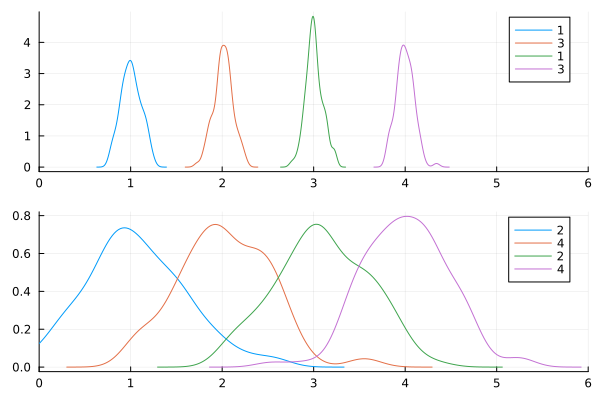# Ordering labels across subplots

Hi,

I would like to add the same label to two subplots. In the example below, the densities and colors are correct, but the label should be `1 2 3 4` for each subplot. Is there a way to correct this issue?

Thanks!

``````using Distributions
using StatsPlots

x = [[rand(Normal(i,.1),100) rand(Normal(i,.5),100)] for i ∈ 1:4]

density(x, layout=(2,1), label=[1 2 3 4], xlims=(0,6))
``````Maybe: `label=[1 1 2 2 3 3 4 4]` ?

2 Likes

That works. Thanks! Maybe a general solution is `repeat(my_labels, inner=n_subplots)'`

Unfortunately, this is not so convenient if `my_label` is non-numeric.

1 Like

You can use `permutedims`:
`permutedims(repeat(my_labels, inner=n_subplots))`

2 Likes